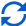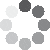Enter parameters Fence
Fence
Fence Length:
ft
• inches (in)
• feet (ft)
• yards (yd)
• centimeters (cm)
• meters (m)
Fence Height:
ft
• inches (in)
• feet (ft)
• yards (yd)
• centimeters (cm)
• meters (m)
Picket Width:
in
• inches (in)
• feet (ft)
• yards (yd)
• centimeters (cm)
• meters (m)
Picket Spacing:
in
• inches (in)
• feet (ft)
• yards (yd)
• centimeters (cm)
• meters (m)
Post Spacing:
ft
• inches (in)
• feet (ft)
• yards (yd)
• centimeters (cm)
• meters (m)
Post Width:
in
• inches (in)
• feet (ft)
• yards (yd)
• centimeters (cm)
• meters (m)
Post Thicknes:
in
• inches (in)
• feet (ft)
• yards (yd)
• centimeters (cm)
• meters (m)
Rails:Result
Number of Posts:
0
Post Height:
0
ft
• inches (in)
• feet (ft)
• yards (yd)
• centimeters (cm)
• meters (m)
Number of Pickets:
0
Number of Rails:
0
Concrete Volume:
0
ft³
• cubic inches (in³)
• cubic feet (ft³)
• cubic yards (yd³)
• cubic centimeters (cm³)
• cubic meters ()(No Ratings Yet)Loading...
Real-time graphics. Make the calculations and see the changes.
Embed this calculator on your site!Add live graphics
Copied to clipboard! PreviewWhat's wrong?
Minimum 5 characters!